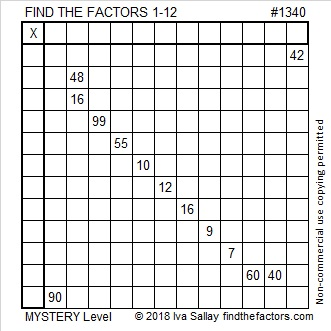# 1340 Mystery Level

Which two of these clues will use both of the 1’s? 10, 12, 9, or 7. I’ll never tell. You will have to solve that mystery yourself!Print the puzzles or type the solution in this excel file: 12 factors 1333-1341

Here are a few facts about the number 1340:

• 1340 is a composite number.
• Prime factorization: 1340 = 2 × 2 × 5 × 67, which can be written 1340 = 2² × 5 × 67
• 1340 has at least one exponent greater than 1 in its prime factorization so √1340 can be simplified. Taking the factor pair from the factor pair table below with the largest square number factor, we get √1340 = (√4)(√335) = 2√335
• The exponents in the prime factorization are 2, 1, and 1. Adding one to each exponent and multiplying we get (2 + 1)(1 + 1)(1 + 1) = 3 × 2 × 2 = 12. Therefore 1340 has exactly 12 factors.
• The factors of 1340 are outlined with their factor pairs in the graphic below.1340 is the hypotenuse of a Pythagorean triple:
804-1072-1340 which is (3-4-5) times 268

This site uses Akismet to reduce spam. Learn how your comment data is processed.